# Engineering Mechanics - General Principles

## Why Engineering Mechanics General Principles?

In this section you can learn and practice Engineering Mechanics Questions based on "General Principles" and improve your skills in order to face the interview, competitive examination and various entrance test (CAT, GATE, GRE, MAT, Bank Exam, Railway Exam etc.) with full confidence.

## Where can I get Engineering Mechanics General Principles questions and answers with explanation?

IndiaBIX provides you lots of fully solved Engineering Mechanics (General Principles) questions and answers with Explanation. Solved examples with detailed answer description, explanation are given and it would be easy to understand. All students, freshers can download Engineering Mechanics General Principles quiz questions with answers as PDF files and eBooks.

## Where can I get Engineering Mechanics General Principles Interview Questions and Answers (objective type, multiple choice)?

Here you can find objective type Engineering Mechanics General Principles questions and answers for interview and entrance examination. Multiple choice and true or false type questions are also provided.

## How to solve Engineering Mechanics General Principles problems?

You can easily solve all kind of Engineering Mechanics questions based on General Principles by practicing the objective type exercises given below, also get shortcut methods to solve Engineering Mechanics General Principles problems.

### Exercise :: General Principles - General Questions

• General Principles - General Questions
1.

Solve the following equation for the two roots of x:   x2 — 16 = 0

 A. x = 2i,   –2i B. x = 4i,   –4i C. x = 4,   –4 D. x = 2,   –2

Explanation:

No answer description available for this question. Let us discuss.

2.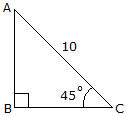Using the basic trigonomic functions, determine the length of side AB of the right triangle.

 A. h = 7.07 B. h = 10 C. h = 5 D. h = 14.14

Explanation:

No answer description available for this question. Let us discuss.

3.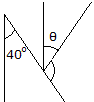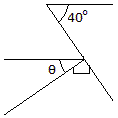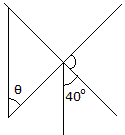Determine the angle:

 A.= 30° B.= 40° C.= 60° D.= 50°

Explanation:

No answer description available for this question. Let us discuss.

4.

Solve the following equation for x, y, and z:

xy + z = –1   –x + y + z = –1   x + 2y – 2z = 5

 A. x = 1,      y = 1,      z = –1 B. x = 5/3,      y = 7/6,      z = –1/2 C. x = –2/3,      y = –2/3,      z = –1 D. x = –1,      y = 1,      z = 1

Explanation:

No answer description available for this question. Let us discuss.

5.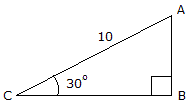Using the basic trigonomic functions, determine the length of side AB of the right triangle.

 A. h = 5.77 B. h = 11.55 C. h = 5 D. h = 8.66